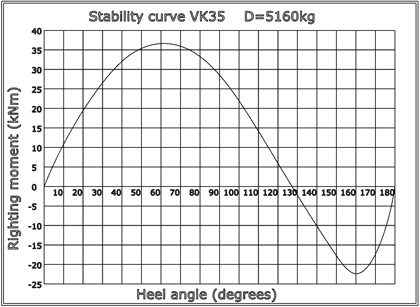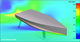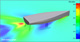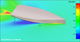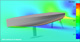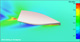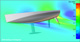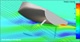Watch dynamic videos

Sails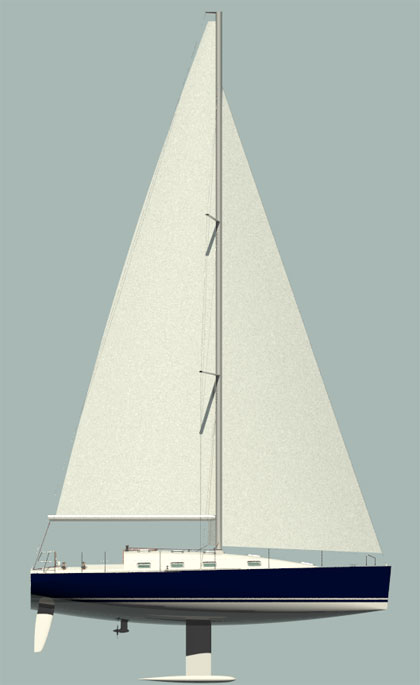VK35 Technical data Length overall = 10,6m Length at waterline = 10,45m Beam overall = 3,5m Beam at waterline = 2,6m Depth at midsection = 3,4m Free board at midsection = 1,3m Draft = 2,17m Displacement = 5160kg Ballast = 1600kg Engine M3.28 20kW (27.2hp) Mainsail area = 38.5m2 Genoa area = 36.7m2 Spinnaker area = 110m2 I = 13.6m J = 4.7m P = 14.5m E = 4.7m Windward balance point at RM30:    RM30 = 27,6kNm    Yaw = 5.3 degrees    V wind = 17.4knots    V hull = 7.4knots Water capacity = 170l Calorifier capacity = 31l Fuel capacity = 88l Gray & black capacity = 45l Boat design category CE: A+ Certification: Ocean plus Design: VK Yacht AB

Stability curve